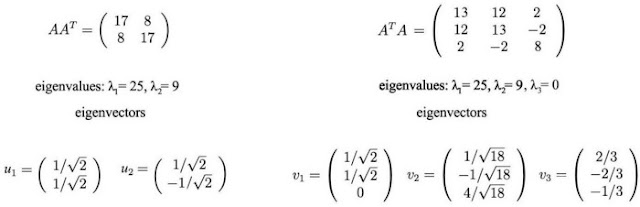Singular Value Decomposition - SVD - Tech It Yourself

## Monday, 12 August 2019

The Singular Value Decomposition states that any matrix A (n x p) can be factored into A = UΣVᵀ where:
- U and V are orthogonal n x n matrices with orthogonal eigenvectors from AAᵀ and AᵀA. Vᵀ is a transposed matrix.
- Σ is a diagonal n x p matrix with the diagonal elements are ordered so that Σii ≥ Σjj for all i < j (descending order). These elements are the root of the positive eigenvalues of AAᵀ or Aᵀ A (AAᵀ and Aᵀ A have the same positive eigenvalues).
- uᵢ and vᵢ have unit length.
Example to find SVD of a matrix:
Applying A to a vector x (Ax = Vᵀx) can be visualized under geometry form.
Vᵀ represents a rotation or reflection of vectors.
Σ represents a linear dilation.
U represents a rotation or reflection.
Figure: geometry form of SVD
Another form of SVD:
σᵢ are descending order so more significant elements left side. Example:We can use SVD for Dimensionality Reduction (just keep the important components). This can be applied when dataset has more features (columns) than observations (rows). This helps reduced dataset to a smaller number of features. If we select the top k largest singular values. An approximate B of the vector A: B = (UΣVᵀ)k
Example:
σ₂ is very small, we can ignore it.
Example: Dataset relation is y=2x, using SVD to reduce dataset.
from numpy import diag
from numpy import zeros
from scipy.linalg import svd
import numpy as np

# define a matrix
A = np.array([[1,2],[2,4],[3,6],[4,8]]).T

# Singular-value decomposition
U, s, VT = svd(A)
# create m x n Sigma matrix
Sigma = zeros((A.shape, A.shape))
# populate Sigma with n x n diagonal matrix
Sigma[:A.shape, :A.shape] = diag(s)
# select
n_elements = 1
Sigma = Sigma[:, :n_elements]
VT = VT[:n_elements, :]
# reconstruct
B = U.dot(Sigma.dot(VT))
print(B)
# transform
T = U.dot(Sigma)
print(T)

Input: [[1. 2. 3. 4.] [2. 4. 6. 8.]]
Output: [[ -5.47722558] [-10.95445115]]